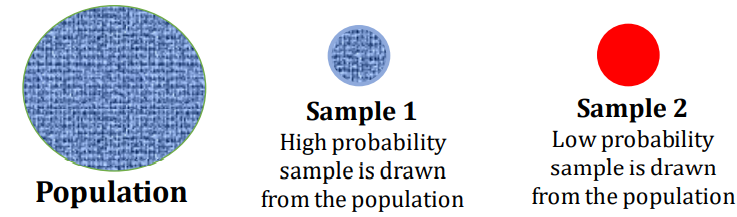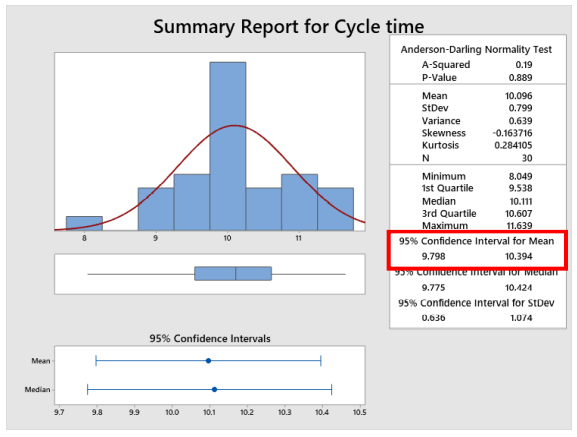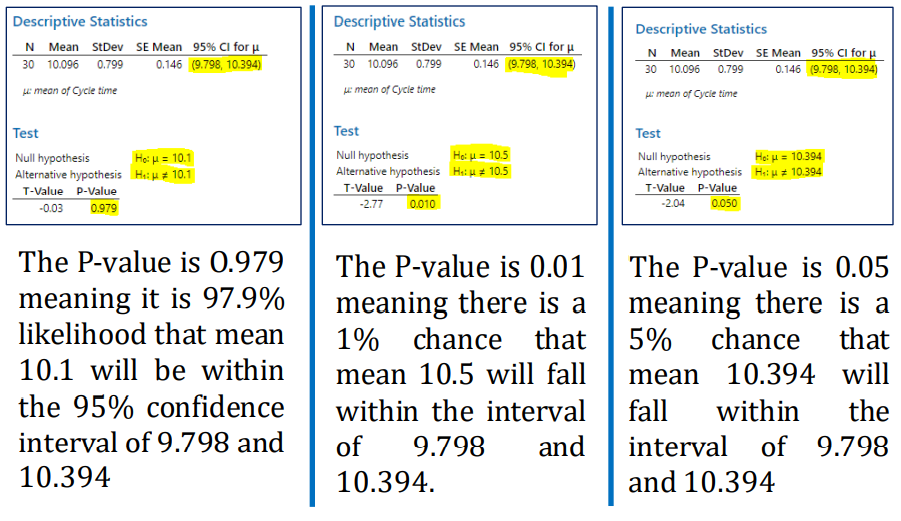# Probability Value (P-Value)• There are many definitions for P-value. Let us decode them one by one
• Definition1:
- Probability (likelihood) is that the samples could have been drawn from the same population being tested
- Explanation: When you consider a sample out of a population, if the sample is drawn from the population, the population parameters and sample statistics will not differ much, and a high probability value (P-value) would indicate the same.
- High P-value such as 0.40 means a 40% chance that the sample is drawn from the population.
- Low P-values say 0.01 means that there is only a 1% chance that sample is drawn from the Population
• Definition 2:
- P-Value is a statistical measure that indicates the estimate of probability of making a type I error.
- Explanation: Let us understand Type I error first, Type I error is rejecting Null hypothesis when it is true, which means that in reality, we should have accepted null hypothesis (there is no difference between the sample and the population) but either due to sampling error or data collection error we are rejecting the null hypothesis
- High P-value such as 0.40 means that if we reject the null hypothesis, there is a 40% chance that we are making a type I error or in other words, the chance of wrongly rejecting the null hypothesis is 40%
- Low P-value, say 0.01, means that there is only a 1% chance that we are wrongly rejecting the null hypothesis
• Definition 3:
- P-Value is Probability of Accepting Null Hypothesis
- Explanation: This one is easy to understand if the Pvalue is 0.4 means that there is a 40% chance of Accepting the null hypothesis
- This one is easy to understand if the P-value is 0.4 means that there is a 40% chance of Accepting the null hypothesis, and a P-value of 0.01 means that there is only a 1% chance of accepting the null hypothesis, so lesser the P-value we can reject the null hypothesis as there is less chance.
• Definition 4:
- The probability that the value being tested falls into a given confidence interval at a defined confidence level.
- For example, let us consider the below graphical summary of a cycle time- We can see that the confidence interval for the mean at 95% confidence level is 9.798 to 10.394. P-value tells you the probability of hypothesized mean value falls within the range of Confidence interval
• Having understood P-value, let us learn about how Statistical decisions are made. Let us understand two terminologies first
• Confidence Level & Significance :
• The confidence we require to make decisions is confidence level. The 95% confidence level means that we need 95% confidence in making decisions (understand through the data). It also means that we are willing to accept a 5% risk which is the significance level.
• Usual significance levels are 0.05, which means we are willing to accept 5% risk by comparing P-value with the significance values the statistical decisions are made
• Let us try to understand by each of the definitions once again
• Definition1:
- Probability (likelihood) is that the samples could have been drawn from the same population being tested
- Now, if the P-value is 0.03 means that there is only a 3% probability that sample is drawn from the population since we are comparing with P-value with 0.05, we can conclude that only 3% chance that sample has drawn from the population, which is lower than the risk that we are willing to accept 5%, and hence we can reject the null hypothesis and conclude data is not drawn from the population
- If P-value is 0.40 means that the Probability of the sample drawn from the population is 40% which is way higher than 5%, we can conclude that we fail to reject the Null hypothesis
• Definition2:
- P-Value is a statistical measure that indicates the probability of making a Type I error.
- Now, if P-value 0.03 means that the probability of making Type I error (wrongly rejecting the null hypothesis) is 3%, and we are willing to accept the risk of 5%, we can say we can go ahead reject the null hypothesis, and we are good to decide that sample is not drawn from the population, and even though we are wrong itis only by 3%
- Now, if P-value 0.40 means that the probability of making Type I error (wrongly rejecting the null hypothesis) is 40%, and we are willing to accept the limit of 5% as 40% is more than 5%, we can conclude that we have a larger risk if we reject the null hypothesiswhich is 40% and we fail to rejectthe null hypothesis
• Definition3:
- P-Value is Probability of Accepting Null Hypothesis
- Now, if P-value 0.03 means that the probability of accepting Null hypothesis is only 3% and we agreed we would accept up to 5% risk and as the probability is only 3% and within our willingness to accept the risk, we can say we reject the null hypothesis
- Now, if P-value 0.40 means that the probability of accepting null hypothesis is 40% which is higher than the limit of 5% we had set, we can conclude we fail to reject the null hypothesis and declare that the Null hypothesis is acceptable
• Definition 4:
- The probability that the value being tested falls into a given confidence interval at a defined confidence level.
- For example, let us consider the below graphical summary of a cycle time
- We can see that the confidence interval for the mean at 95% confidence level is 9.798 to 10.394. Now let us test 3 hypothesized means and find out how our P-value changes.
- If we test new means within the confidence interval, the Pvalue will be more than 0.05, and if we test the new mean outside confidence interval P-value will be less than 0.05, and right on the confidence interval, P-value would be exactly 0.05. Let us test it with one Sample T-Test
• At 95% confidence level, we can say that mean would be anywhere between 9.798 to 10.394 and let us test 3 means one exactly at the mean, one away from Confidence interval and one right on the confidence interval and see how P-value changesTOP